# Math graph for Android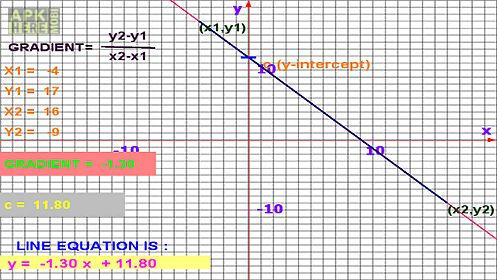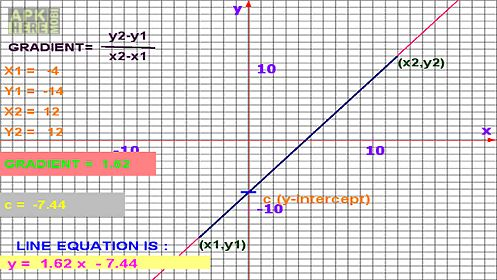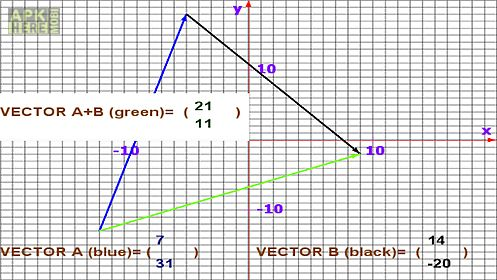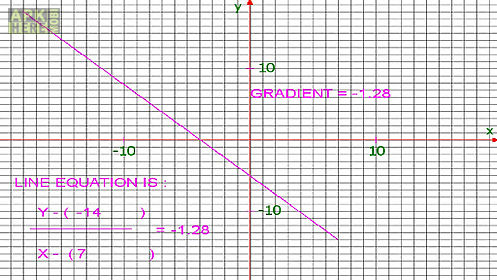Math graph app for android description: This app will help beginners in graphical methods understand the linear equation y mx+c.
Learn about linear graphs and vectors simply by just a swipe.
Select graphs or vectors on intro page.
Find the relationship between lines gradients y intercept linear equations and vectors by drawing a graph with your finger while this app does the rest.
No input of values is necessary.
This is an automatic graph app.
Math graph app you draw graph it works out the equation for you.
Trace the graph with your finger.
When done sit back and study your graph.
The app will draw the line and calculate gradient.
It will also write down the graph equation at bottom left of screen.
On vectors screen you draw vectors with your finger and this app analyzes them for you.
It is an easy way to learn about simple vectors including addition of vectors.
This native math app is meant for beginners in graphical methods the first 2 years of learning graphs.
If you have studied complex graphical methods but would still like to remind yourself about the basics this app will do just that.
You can work with the equations produced at your leisure re arranging them to discover more about graphical mathematics.

## Related Apps to Math graph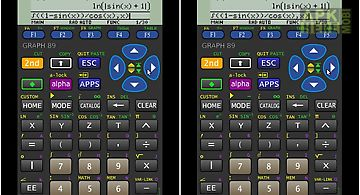Graph 89 free
Graph 89 emulator for the ti 89 graphing calculator. The rom image is not included. You need to extract this from you own calculator. Make sure you have th..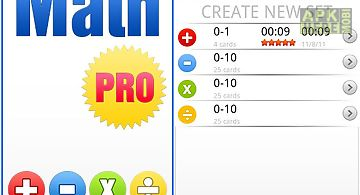Math pro - math game for kids
math pro math game for kids by studios parents and students have asked for a more advance version of our very popular math practice flash cards app and he..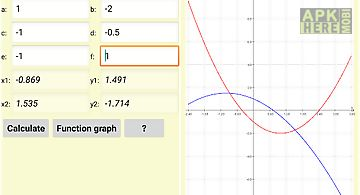Math expert
The application math expert is a collection of formulas out of mathematics and physics. The special feature is that the application can calculate the formu..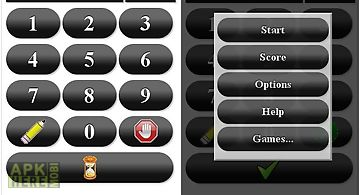Math tutor
Tired of math tutoring that are more of a game than tutoring? You are at the right spot. We don t have cute farmville pets or angry birds. Instead we are s..Capture math
Capture math beta release. Final release soon. Take a snap of the printed expression and within a blink get the answer. Besides capturing you can also ente..All math formula
Here is maths formulas pack for all android users. This app has 1000+ math formula and more to come. Now no need to make paper notes to remember mathematic..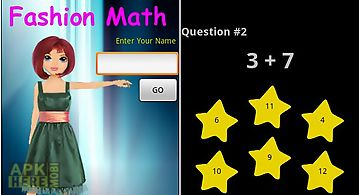Fashion math
The highly rated fashion math game designed especially for girls previously available only on the iphone now available for the android. Fashion math is one..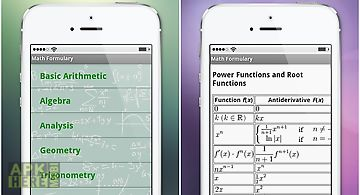Math formulary
One of the most powerful and incredible creations of the human mind from galileo to einstein is now available for you on your smartphone. Thanks to our app..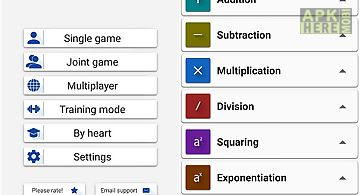Math tricks
This program is designed for those who want to learn interesting mathematical tricks to speed up the calculating. These tricks will help solve part of the ..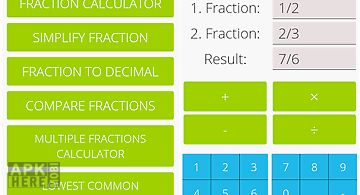Fractions math
This free math app is a fractions calculator with various functions: add subtract multiply and divide fractions. Convert fractions in decimals simplify fra..Math for kids
Math for kids is an educational game which teaches kids the basic math to learn number develops kids learning number skills. Christmas is coming let yourki..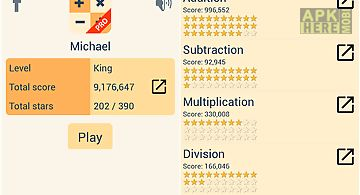King of math
1 math app on google play be king of math secret math formulas 100s of challenges await youaddition subtraction fraction equalities geometrics statistics ..
Apktidy.com - Download free math graph.apk for Android 2.3+ mobile. ApkHere is really the best place to quickly find thousands useful apk apps and new android video games for Samsung Galaxy, Huawei, LG, Sony, Meizu, Asus android phone or tablet devices. All Apk Here apps sort of the most popular categories like education, photo editor, social, fitness, tools apps and brain, card, action, puzzle, online, arcade, racing games in .apk files format. Simple, Fast and safe Apk apps store for your android at Apktidy.com